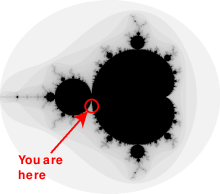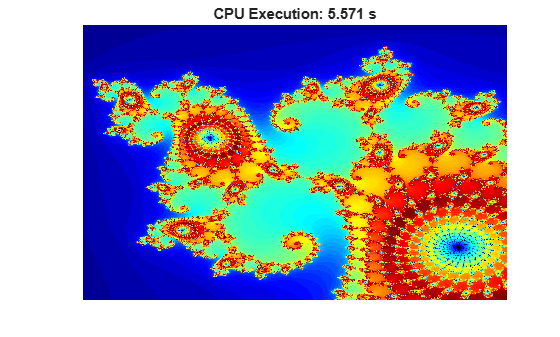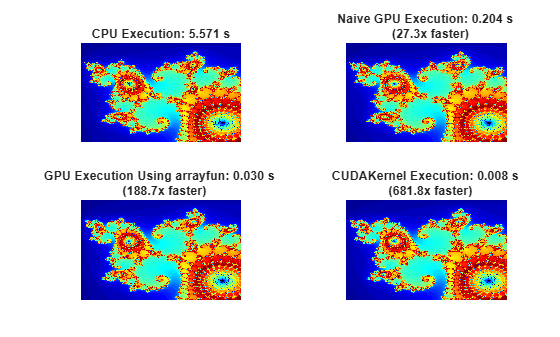Illustrating Three Approaches to GPU Computing: The Mandelbrot Set

This example shows how a simple, well-known mathematical problem, the Mandelbrot Set, can be expressed in MATLAB® code. Using Parallel Computing Toolbox™ this code is then adapted to make use of GPU hardware in three ways:

1. Using the existing algorithm but with GPU data as input

2. Using arrayfun to perform the algorithm on each element independently

3. Using the MATLAB/CUDA interface to run some existing CUDA/C++ code

Setup

The values below specify a highly zoomed part of the Mandelbrot Set in the valley between the main cardioid and the p/q bulb to its left.A 1000x1000 grid of real parts (X) and imaginary parts (Y) is created between these limits and the Mandelbrot algorithm is iterated at each grid location. For this particular location 500 iterations will be enough to fully render the image.

maxIterations = 500;
gridSize = 1000;
xlim = [-0.748766713922161, -0.748766707771757];
ylim = [ 0.123640844894862,  0.123640851045266];

The Mandelbrot Set in MATLAB

Below is an implementation of the Mandelbrot Set using standard MATLAB commands running on the CPU. This is based on the code provided in Cleve Moler's "Experiments with MATLAB" e-book.

This calculation is vectorized such that every location is updated at once.

% Setup
t = tic();
x = linspace( xlim(1), xlim(2), gridSize );
y = linspace( ylim(1), ylim(2), gridSize );
[xGrid,yGrid] = meshgrid( x, y );
z0 = xGrid + 1i*yGrid;
count = ones( size(z0) );

% Calculate
z = z0;
for n = 0:maxIterations
z = z.*z + z0;
inside = abs( z )<=2;
count = count + inside;
end
count = log( count );

% Show
cpuTime = toc( t );
fig = gcf;
fig.Position = [200 200 600 600];
imagesc( x, y, count );
colormap( [jet();flipud( jet() );0 0 0] );
axis off
title( sprintf( '%1.2fsecs (without GPU)', cpuTime ) );Using gpuArray

When MATLAB encounters data on the GPU, calculations with that data are performed on the GPU. The class gpuArray provides GPU versions of many functions that you can use to create data arrays, including the linspace, logspace, and meshgrid functions needed here. Similarly, the count array is initialized directly on the GPU using the function ones.

With these changes to the data initialization the calculations will now be performed on the GPU:

% Setup
t = tic();
x = gpuArray.linspace( xlim(1), xlim(2), gridSize );
y = gpuArray.linspace( ylim(1), ylim(2), gridSize );
[xGrid,yGrid] = meshgrid( x, y );
z0 = complex( xGrid, yGrid );
count = ones( size(z0), 'gpuArray' );

% Calculate
z = z0;
for n = 0:maxIterations
z = z.*z + z0;
inside = abs( z )<=2;
count = count + inside;
end
count = log( count );

% Show
count = gather( count ); % Fetch the data back from the GPU
naiveGPUTime = toc( t );
imagesc( x, y, count )
axis off
title( sprintf( '%1.3fsecs (naive GPU) = %1.1fx faster', ...
naiveGPUTime, cpuTime/naiveGPUTime ) )Element-wise Operation

Noting that the algorithm is operating equally on every element of the input, we can place the code in a helper function and call it using arrayfun. For GPU array inputs, the function used with arrayfun gets compiled into native GPU code. In this case we placed the loop in pctdemo_processMandelbrotElement.m:

function count = pctdemo_processMandelbrotElement(x0,y0,maxIterations)
z0 = complex(x0,y0);
z = z0;
count = 1;
while (count <= maxIterations) && (abs(z) <= 2)
count = count + 1;
z = z*z + z0;
end
count = log(count);

Note that an early abort has been introduced because this function processes only a single element. For most views of the Mandelbrot Set a significant number of elements stop very early and this can save a lot of processing. The for loop has also been replaced by a while loop because they are usually more efficient. This function makes no mention of the GPU and uses no GPU-specific features - it is standard MATLAB code.

Using arrayfun means that instead of many thousands of calls to separate GPU-optimized operations (at least 6 per iteration), we make one call to a parallelized GPU operation that performs the whole calculation. This significantly reduces overhead.

% Setup
t = tic();
x = gpuArray.linspace( xlim(1), xlim(2), gridSize );
y = gpuArray.linspace( ylim(1), ylim(2), gridSize );
[xGrid,yGrid] = meshgrid( x, y );

% Calculate
count = arrayfun( @pctdemo_processMandelbrotElement, ...
xGrid, yGrid, maxIterations );

% Show
count = gather( count ); % Fetch the data back from the GPU
gpuArrayfunTime = toc( t );
imagesc( x, y, count )
axis off
title( sprintf( '%1.3fsecs (GPU arrayfun) = %1.1fx faster', ...
gpuArrayfunTime, cpuTime/gpuArrayfunTime ) );Working with CUDA

In Experiments in MATLAB improved performance is achieved by converting the basic algorithm to a C-Mex function. If you are willing to do some work in C/C++, then you can use Parallel Computing Toolbox to call pre-written CUDA kernels using MATLAB data. You do this with the parallel.gpu.CUDAKernel feature.

A CUDA/C++ implementation of the element processing algorithm has been hand-written in pctdemo_processMandelbrotElement.cu: This must then be manually compiled using nVidia's NVCC compiler to produce the assembly-level pctdemo_processMandelbrotElement.ptx (.ptx stands for "Parallel Thread eXecution language").

The CUDA/C++ code is a little more involved than the MATLAB versions we have seen so far, due to the lack of complex numbers in C++. However, the essence of the algorithm is unchanged:

__device__
unsigned int doIterations( double const realPart0,
double const imagPart0,
unsigned int const maxIters ) {
// Initialize: z = z0
double realPart = realPart0;
double imagPart = imagPart0;
unsigned int count = 0;
// Loop until escape
while ( ( count <= maxIters )
&& ((realPart*realPart + imagPart*imagPart) <= 4.0) ) {
++count;
// Update: z = z*z + z0;
double const oldRealPart = realPart;
realPart = realPart*realPart - imagPart*imagPart + realPart0;
imagPart = 2.0*oldRealPart*imagPart + imagPart0;
}
return count;
}

One GPU thread is required for location in the Mandelbrot Set, with the threads grouped into blocks. The kernel indicates how big a thread-block is, and in the code below we use this to calculate the number of thread-blocks required. This then becomes the GridSize.

cudaFilename = 'pctdemo_processMandelbrotElement.cu';
ptxFilename = ['pctdemo_processMandelbrotElement.',parallel.gpu.ptxext];
kernel = parallel.gpu.CUDAKernel( ptxFilename, cudaFilename );

% Setup
t = tic();
x = gpuArray.linspace( xlim(1), xlim(2), gridSize );
y = gpuArray.linspace( ylim(1), ylim(2), gridSize );
[xGrid,yGrid] = meshgrid( x, y );

% Make sure we have sufficient blocks to cover all of the locations
numElements = numel( xGrid );

% Call the kernel
count = zeros( size(xGrid), 'gpuArray' );
count = feval( kernel, count, xGrid, yGrid, maxIterations, numElements );

% Show
count = gather( count ); % Fetch the data back from the GPU
gpuCUDAKernelTime = toc( t );
imagesc( x, y, count )
axis off
title( sprintf( '%1.3fsecs (GPU CUDAKernel) = %1.1fx faster', ...
gpuCUDAKernelTime, cpuTime/gpuCUDAKernelTime ) );Summary

This example has shown three ways in which a MATLAB algorithm can be adapted to make use of GPU hardware:

1. Convert the input data to be on the GPU using gpuArray, leaving the algorithm unchanged

2. Use arrayfun on a gpuArray input to perform the algorithm on each element of the input independently

3. Use parallel.gpu.CUDAKernel to run some existing CUDA/C++ code using MATLAB data

title('The Mandelbrot Set on a GPU')Parallel Computing Toolbox DocumentationGet trial now